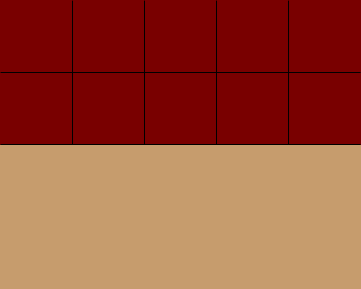SEARCH HOMEMath Central Quandaries & QueriesQuestion from jane: if a room is 4meters by 5meters, how many square meters are there?Hi Jane,

Imagine that you want to tile the floor using tiles that are 1 metre by 1 metre. Each tile has an area of 1 square metre. You can put 5 tiles along the long wall and then 5 more tiles beside the first row and so on.When you are done you have 4 rows of 5 tiles each for a total of 4 × 5 = 20 tiles and hence 20 square metres.

PennyMath Central is supported by the University of Regina and The Pacific Institute for the Mathematical Sciences.